# Transformer Cheat Sheet

·        A transformer is used to transform from one voltage to another.

·        The voltage ratio is proportional to the turns ration.  Current is inversely proportional to the turns ratio.

·        The power ratio of an ideal transformer is 1:1.  In other words, if the voltage goes up, the current must go down.

·        Transformers only work with AC, Alternating Current.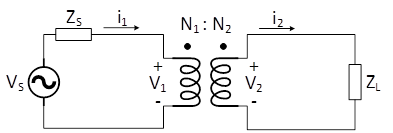## Dots and phase

·       The dots can be thought of as voltage polarity.

·       When current flows into the dot on the primary side, an induced current flows out of the dot on the secondary.

·       The voltage is in phase at the dots, but the current is flowing in the opposite direction.

## Impedance Matching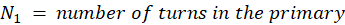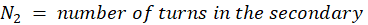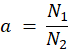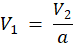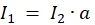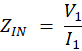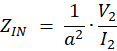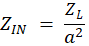## Common Mode Choke

When configured as a common mode choke, a transformer is seen as a low impedance to differential current and high impedance to common mode current.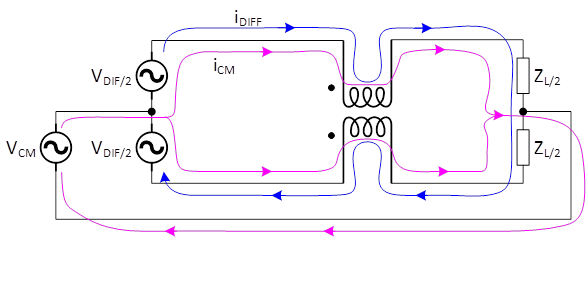·        When common mode current flows through the windings, the flux adds, creating more inductance.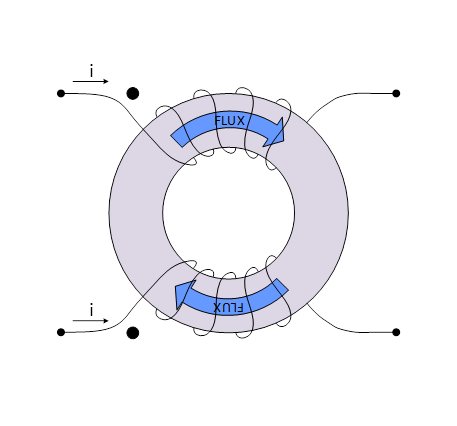·        When differential current flows through the windings, the flux cancels, reducing the inductance.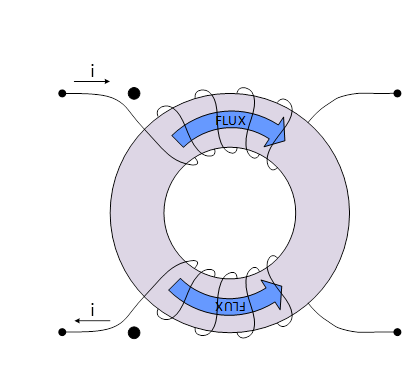end